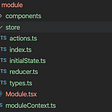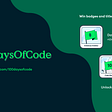# Learning to Code: Day 53 — Basic JavaScript Part 18

Hello all! A fine good evening to you tonight, let’s get back into this JavaScript business then shall we? Let’s finish up on a bit more Recursion for today, keeping it short and sweet. Cheers to FreeCodeCamp for the lessons.

## Using Recursion to create a Countdown

If like me you’ve always wanted to create a countdown with Recursion through JavaScript, well you’ve come to the right place buddy!

We now know how to use recursion instead of a for loop, now let’s look at a function which by using recursion, returns a series of integers into an array, starting from 1.

Firstly we need a base case: Where the return value is already known and the recursive function no longer needs to call itself.

Secondly, we need a recursive call: This executes the original function with different arguments each time. To paraphrase FreeCodeCamp here: if written correctly, “the base case is eventually reached”.

So, let’s say you want to create a function which returns a series of integers into an array, starting from 1 until n, the function will need to accept an argument which states that number n is the last stop in the recursion. From there, it needs to recall itself in progressively smaller numbers of n until it reaches 1. Let’s take a look at that:

Note: The keyword “const” means “constant”, as in, what I write/define next will not be modified later on. This is different to var as var definitions can be modified and adjusted later on, which can cause bug problems in larger code.

Spot the obvious here? The array is increasing, however the recursive function is decreasing, this outcome is due to the fact that .push() happens after each recursive call has returned. At the moment that n is pushed into the array, (n-1) has already been evaluated and returned.. [1,2,3,..n-1]. So I think we can say that .push() is happening slower than the recursion is taking effect.

But what if we want to do this in reverse and have a descending array? Well, we can change a small part of this code example above, where the array was ascending and .push() put the numbers on the end, let’s use .unshift() (note that change on line 6 below) to “push” the numbers on the beginning, this way, we’ll have a descending array:

## Creating a Range with Recursion

If we have two perimeters in the function instead of one (n on line 1 above), how would this change the outlook of the function?

Let’s say we want to specify a range between point A and point B, instead of defining a predefined starting point of 1 up to n, like above. Let’s look at the code first then break it down:

So on line 1 we have our two perimeters - the starting point startNum and ending point endNum. Those are our two perimeters that were working inside of. If the user inputs two equal numbers, we want the array to output that single number (this is outlined in lines 2 and 3). Line 2 says “If the starting number minus the ending number is strictly equal to 0, then return the second number” (or the starting number, it wouldn’t matter, as they are the same in this case).

Next, from line 4 in the else statement, we say “otherwise, take a variable called numbers which takes the recursive call of (startNum, endNum - 1), and push that new endNum onto the end of the array”. This is effectively saying, take the higher number (endNum), decrease it by 1, and add that new value into the array sequence as each recursion occurs. The result of which is an increasing array from point A (startNum) to point B (endNum) and all the whole integers in-between.

Aaaaaaaand let’s keep it short today. Thanks for checking in! See you next time for more on JavaScript.

--

--

--

## More from The Startup

Get smarter at building your thing. Follow to join The Startup’s +8 million monthly readers & +756K followers.

## Creating Console Signatures in Javascript## vue.js: from internationalization (i18n) to localization (l10n) and back again## A simple calculator using HTML, CSS and JavaScript## State management with React Hooks in TypeScript## Day15 of #100DaysOfCode## Evaluating Time Complexity of Native JavaScript Methods## Material Design Motions with React Native — Hello world## Solution for all the SelectorsHub issues like not able to find SelectorsHub in devtools, xpath not…## Hugh Burgess

Step by step, learning to code with FreeCodeCamp

## Why You Should Learn Programming?## 5 Resources that shaped my understanding of development## What is Big O Notation? And why do I need to know it to get a Software Engineer job?